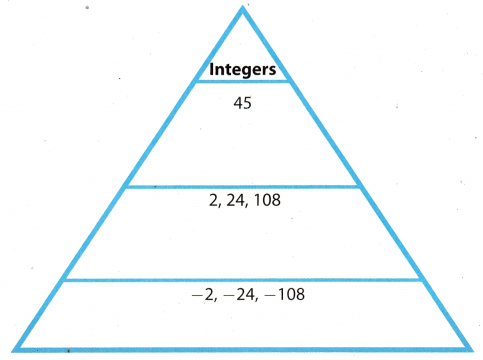# Texas Go Math Grade 7 Module 1 Answer Key Rational Numbers

Refer to our Texas Go Math Grade 7 Answer Key Pdf to score good marks in the exams. Test yourself by practicing the problems from Texas Go Math Grade 7 Module 1 Answer Key Rational Numbers.

## Texas Go Math Grade 7 Module 1 Answer Key Rational Numbers

Multiply. Write the product in simplest form.

Question 1.
$$\frac{9}{14}$$ × $$\frac{7}{6}$$ ____________
$$\frac{9}{14}$$ × $$\frac{7}{6}$$
= $$\frac{3}{4}$$

Question 2.
$$\frac{3}{5}$$ × $$\frac{4}{7}$$ ____________
We do not have any common factors here to divide with.
Simplify:
= $$\frac{12}{35}$$

Question 3.
$$\frac{11}{8}$$ × $$\frac{10}{33}$$ ____________
$$\frac{11}{8}$$ × $$\frac{10}{33}$$
= $$\frac{5}{12}$$

Question 4.
$$\frac{4}{9}$$ × 3 ____________
Write 3 as $$\frac{3}{1}$$
$$\frac{4}{9}$$ × $$\frac{3}{1}$$
= $$\frac{4}{3}$$

Divide.

Question 5.
$$\frac{1}{2}$$ ÷ $$\frac{1}{4}$$ ____________
= $$\frac{1}{2}$$ × $$\frac{4}{1}$$ Multiply by the reciprocal of the divisor.
= $$\frac{1}{2}$$ × $$\frac{4}{1}$$ Divide by the common factors.
= $$\frac{2}{1}$$ = 2 Simplify
= 2

Question 6.
$$\frac{3}{8}$$ ÷ $$\frac{13}{16}$$ ____________
= $$\frac{3}{8}$$ × $$\frac{16}{13}$$ Multiply by the reciprocal of the divisor.
= $$\frac{3}{8}$$ × $$\frac{16}{12}$$ Divide by the common factors.
= $$\frac{6}{13}$$ Simplify
= $$\frac{6}{13}$$

Question 7.
$$\frac{2}{5}$$ ÷ $$\frac{14}{15}$$ ____________
= $$\frac{2}{5}$$ × $$\frac{15}{14}$$ Multiply by the reciprocal of the divisor.
= $$\frac{2}{5}$$ × $$\frac{15}{15}$$ Divide by the common factors.
= $$\frac{3}{7}$$ Simplify
= $$\frac{3}{7}$$

Question 8.
$$\frac{4}{9}$$ ÷ $$\frac{16}{27}$$ ____________
= $$\frac{4}{9}$$ × $$\frac{27}{16}$$ Multiply by the reciprocal of the divisor.
= $$\frac{4}{9}$$ × $$\frac{27}{16}$$ Divide by the common factors.
= $$\frac{3}{4}$$ Simplify
= $$\frac{3}{4}$$

Question 9.
$$\frac{3}{5}$$ ÷ $$\frac{5}{6}$$ ____________
= $$\frac{3}{5}$$ × $$\frac{6}{5}$$ Multiply by the reciprocal of the divisor.
= $$\frac{18}{25}$$ No common factors. Simplify
= $$\frac{18}{25}$$

Question 10.
$$\frac{1}{4}$$ ÷ $$\frac{23}{24}$$ ____________
= $$\frac{1}{4}$$ × $$\frac{24}{23}$$ Multiply by the reciprocal of the divisor.
= $$\frac{1}{4}$$ × $$\frac{24}{23}$$ Divide by the common factors.
= $$\frac{6}{23}$$ Simplify
= $$\frac{6}{23}$$

Question 11.
6 ÷ $$\frac{3}{5}$$ ____________
Write 6 as $$\frac{6}{1}$$
= $$\frac{6}{1}$$ × $$\frac{5}{3}$$ Multiply by the reciprocal of the divisor.
= $$\frac{6}{1}$$ × $$\frac{5}{3}$$ Divide by the common factors.
= $$\frac{10}{1}$$ = 10 Simplify
= 10

Question 12.
$$\frac{4}{5}$$ ÷ 10 ____________
Write 10 as $$\frac{10}{1}$$
= $$\frac{4}{5}$$ × $$\frac{10}{1}$$ Multiply by the reciprocal of the divisor.
= $$\frac{4}{5}$$ × $$\frac{10}{1}$$ Divide by the common factors.
= $$\frac{8}{1}$$ = 8 Simplify
= 8

Evaluate each expression.

Question 13.
21 – 6 ÷ 3 _________
= 21 – 2 MultipLy and divide from Left to right.
= 19 Add and substract from left to right
= 19

Question 14.
18 + (7 – 4) × 3 _________
If first step get rid of parentheses to obtain:
18 + (7 – 3) • 3 = 18 + 3 • 3
In second step multiply from left to right.
18 + 3 • = 18 + 9
Third step is where we add from left to right.
18 + 9 = 27
= 27

Question 15.
5 + (8 – 3)2 ___________
= 5 + (5)2 Operate within parenthesis.
= 5 + 25 SimpLify exponents
= 30 Add and substract from Left to right.
= 30

Question 16.
9 + 18 ÷ 3 + 10 ________
= 9 + 6 + 10 MuLtiply and divide from Left to right
= 15 + 10 Add and substract from Left to right.
= 25

Question 17.
60 – (3 – 1)4 × 3 _________
= 60 – (2)4 × 3 Operate within parenthesis.
= 60 – 16 × 3 SimpLify exponents
= 60 – 48 MuLtiply and divide from Left to right
= 12 Add and substract from left to right.
= 12

Question 18.
10 – 16 ÷ 4 × 2 + 6 _________
= 10 – 4 × 2 + 6 MuLtipLy and divide from Left to right
= 10 – 8 + 6 Add and substract from Left to right.
= 2 + 6
= 8

Visualize Vocabulary
Use the ✓ words to complete the graphic. You can put more than one word in each section of the triangle.Understand Vocabulary

Complete the sentences using the preview words.

Question 1.
A group of items is a __________. A set contained within another set is a __________.